More on Potentiometers to Attenuate a Loudspeaker

and some of the effects on impedance and crossover point

An addendum to an earlier page

 PHOTO 1 The pot used here, a Honeywell 53C350. It's wirewound, 50 ohms and rated at 2 watts. This unit measures 52 ohms. The pin references made in this page are: 1, 2, 3 from left to right.The tweeter used in this study was a Wharfedale Super 3 with a rated impedance of 10 to 15 ohms.  Its actual impedance at 5 khz is 11.4 ohms.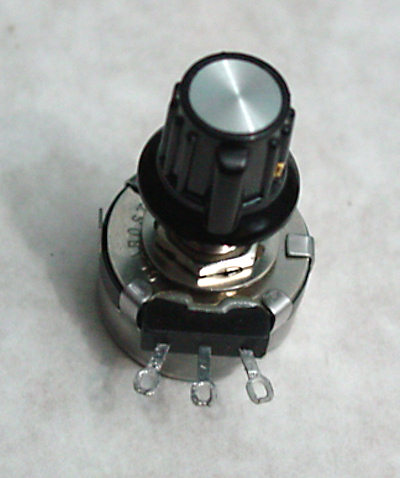FIG. 1 This is just a reference schematic.  Rs and Rp are the parts of the potentiometer wired in series and parallel with the speaker (load).  The numbers 3, 2 and 1 from left to right on the sawtooth (resistor) refer to the terminals on same.   Below that, the numbers 5, 4, 3, 12, 9 and 7 refer to clock positions from clockwise (CW) to counter clockwise (CCW). The C (on top) is the high pass filter and it doesn't matter if it's wired at the input or the output but my preference would be the input despite the drawing.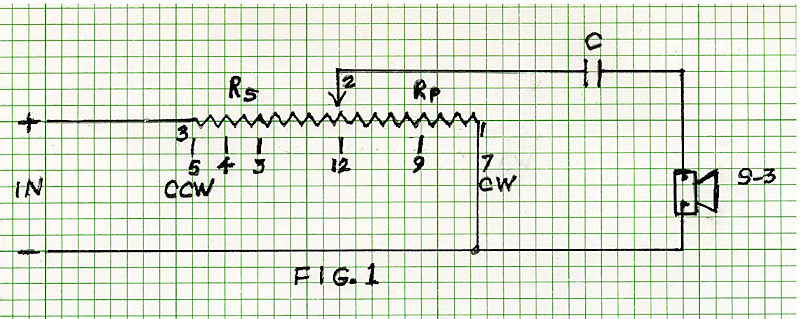FIG. 2 Seems I forgot to number the potentiometer.  To the left of Rs 26 is 3, the junction between Rs and Rp is 2 and the bottom of Rp 26 is 1. The 11.4 ohms is the impedance of the Super 3 at 5000hz. This schematic above shows the potentiometer at 12 o'clock, midway.  We have a 26 ohms resistor in parallel with the speaker and this pair is in series with Rs 26. The effective load imposed on the amplifier is now 33.9 ohms at 5khz.The attenuation of the tweeter with the pot in this position (12 o'clock) is about 12dBSee the red(15) & blue(12) curves in FIG. 6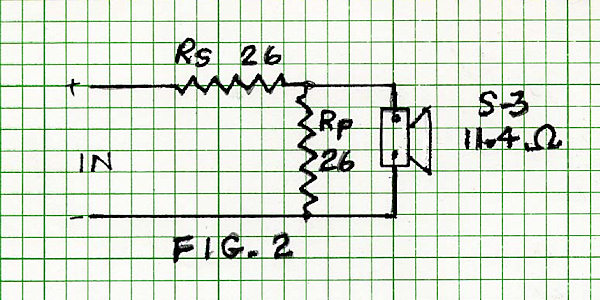FIG. 3 This is an alternate way to represent the schematic of Fig.2.  They are electrically identical but this arrangement was considered easier to see the Rp & S3 parallel pair being in series with Rs.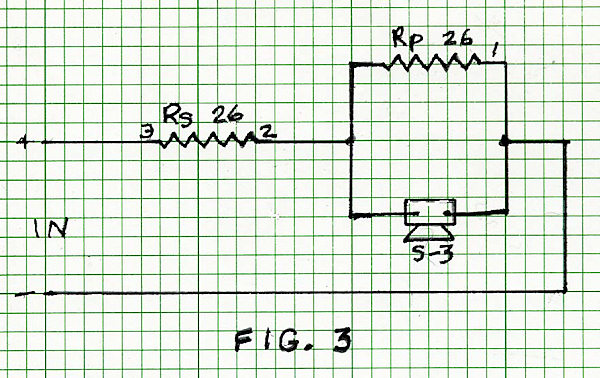FIG. 4 The potentiometer, hereafter referred to as 'the pot' is shown in full clockwise position.  The tweeter would be at full output, no significant attenuation.  The effective load imposed on the amplifier is now 9.35 ohms at 5khz. In this position, 18% of the power is delivered to the resistor.  (see black & red curves FIG. 5, a loss of about 2dB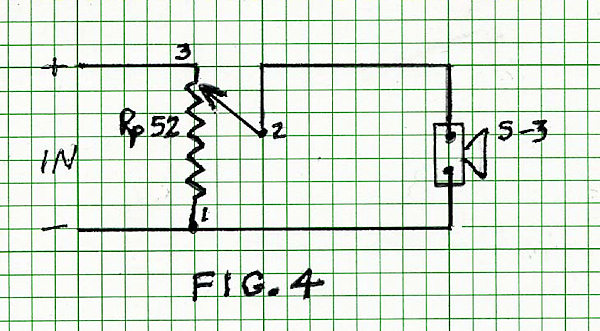Impedance plots with the pot at various positions, CCW(7), 9, 12(noon), 3, 4 and CW(5)

The black curve, #2 is that of the tweeter without the pot connected.

The red curve, #8 is with the pot at full CW (5 o'clock) position.  This curve is 2dB below the black one due to the loss imposed by the 52 ohms of the pot in parallel with the tweeter.

The blue curve (3 on top) is with the pot fully CCW.  The tweeter is now shorted and the amplifier sees only the 52 ohms resistor.

FIG. 5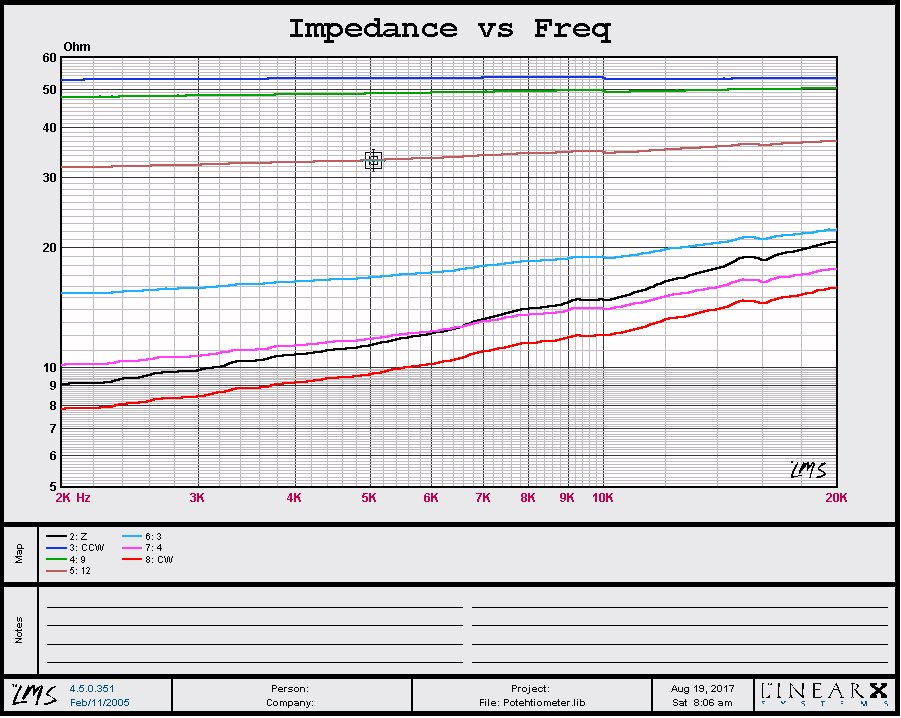SPL measured at about 0.25m.  Input power was arbitrary as this was to show how the filter slope changes with different pot positions which affects impedance and thus the crossover point. The purple curve (16) on top is with no capacitor and the turquoise one (between red & black) is with a 3uF cap and no pot.  The red (15) almost superimposed on the turquoise is with the pot and cap and the pot is at 5 o'clock, full CW.  This puts the 52 ohms in parallel with the tweeter thus changing the impedance to 9.35 ohms at 5khz.  The 2 ohms difference doesn't seem to make any appreciable difference in the slope.

The convergence of the curves as the frequency drops is most likely due to the impedance of the tweeter approaching its dc resistance (7.6 ohms)

FIG. 6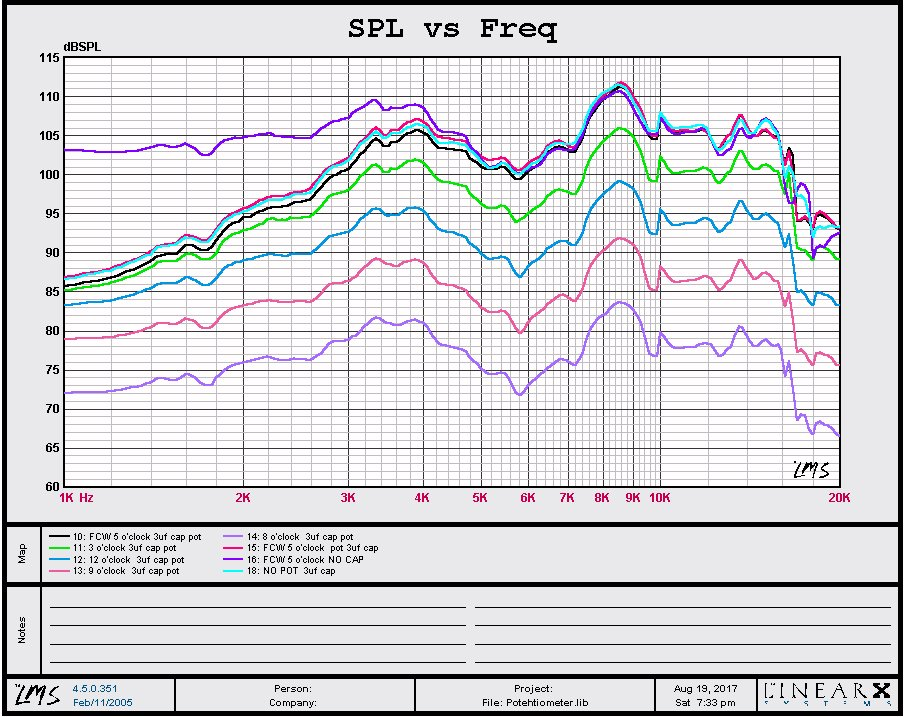Response difference between using a 2uf (green) and 3uf (red) high pass filter.  The system impedance here is 9.6 ohms with the pot FCW (5 o'clock)

If memory serves me well, the W3 uses a 2uf as probably does the W2 and W4 as they all use the same Super 3 tweeter.  The sonic difference isn't worthy of mention or argument.

FIG. 7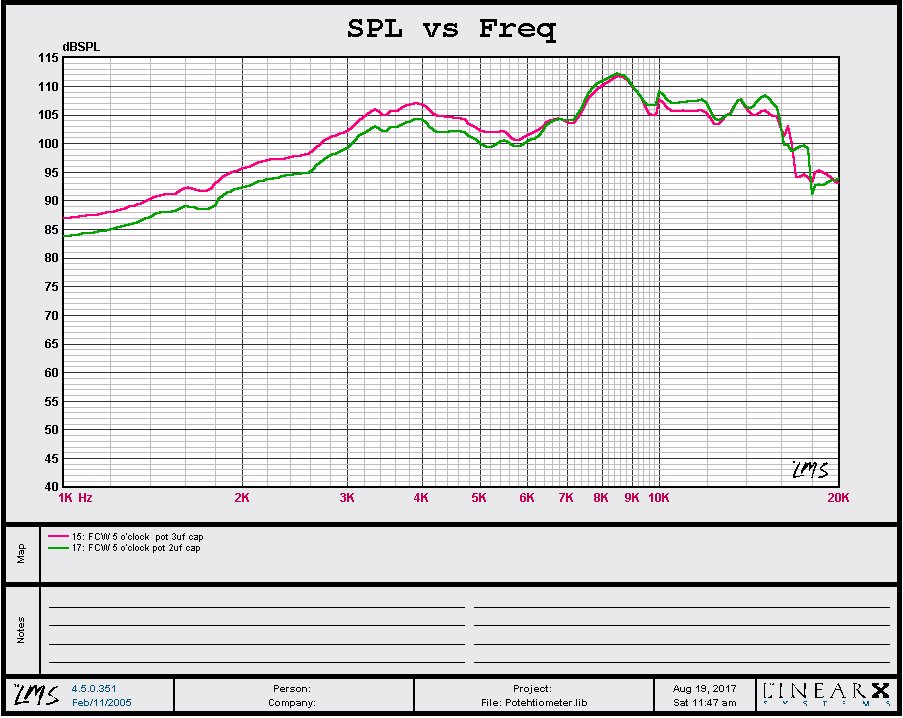Back to the loudspeaker main page